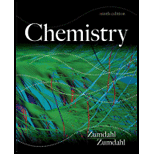# What is a hydrocarbon? What is the difference between a saturated hydrocarbon and an unsaturated hydrocarbon? Distinguish between normal and branched hydrocarbons. What is an alkane? What is a cyclic alkane? What are the two general formulas for alkanes? What is the hybridization of carbon atoms in alkanes? What are the bond angles in alkanes? Why are cyclopropane and cyclobutane so reactive? The normal (unbranched) hydrocarbons are often referred to as straight-chain hydrocarbons. What does this name refer to? Does it mean that the carbon atoms in a straight-chain hydrocarbon really have a linear arrangement? Explain. In the shorthand notation for cyclic alkanes, the hydrogens are usually omitted. How do you determine the number of hydrogens bonded to each carbon in a ring structure?### Chemistry

9th Edition
Steven S. Zumdahl
Publisher: Cengage Learning
ISBN: 9781133611097### Chemistry

9th Edition
Steven S. Zumdahl
Publisher: Cengage Learning
ISBN: 9781133611097

#### Solutions

Chapter
Section
Chapter 22, Problem 1RQ
Textbook Problem

## What is a hydrocarbon? What is the difference between a saturated hydrocarbon and an unsaturated hydrocarbon? Distinguish between normal and branched hydrocarbons. What is an alkane? What is a cyclic alkane? What are the two general formulas for alkanes? What is the hybridization of carbon atoms in alkanes? What are the bond angles in alkanes? Why are cyclopropane and cyclobutane so reactive?The normal (unbranched) hydrocarbons are often referred to as straight-chain hydrocarbons. What does this name refer to? Does it mean that the carbon atoms in a straight-chain hydrocarbon really have a linear arrangement? Explain. In the shorthand notation for cyclic alkanes, the hydrogens are usually omitted. How do you determine the number of hydrogens bonded to each carbon in a ring structure?

Expert Solution
Interpretation Introduction

Interpretation: The answers of various questions related to hydrocarbons are to be stated.

Concept introduction: The organic compounds containing carbon and hydrogen are known as hydrocarbons. They can be further classified as saturated and unsaturated hydrocarbons. Saturated hydrocarbons contain only single bond between atoms while unsaturated hydrocarbons contains double or triple bonds between the atoms of the molecule.

The given questions have been rightfully answered.

### Explanation of Solution

Explanation

To explain: The answers of various questions related to hydrocarbons.

Hydrocarbons are the simplest organic compounds.

The organic compounds containing carbon and hydrogen are known as hydrocarbons. They can be straight chain, branched chain or cyclic molecules.

The difference between saturated and unsaturated hydrocarbons is that saturated hydrocarbons contain only single bonds between atoms while unsaturated hydrocarbons contain double or triple bonds between the atoms.

In normal straight-chain hydrocarbons, carbon atoms form long chains while in branched hydrocarbons, hydrogen attached to carbon is substituted by any other group.

When the hydrocarbons contain only single bonds, then they are known as alkanes. The general formula of alkanes is CnH2n+2 . Alkanes having one or more ring of carbon atoms is present in their structure are known as cycloalkanes. Their general formula is CnH2n .

The hybridization of carbon atom in alkanes is sp3 .

All the carbon atoms in alkanes are joined by single bonds, thus its hybridization is sp3 .

In alkanes, each carbon atom forms four bonds and each hydrogen atom forms a single bond with a carbon atom. The bonding around each carbon is tetrahedral, therefore all the bond angles are 109.5°

The carbon atoms in cyclopropane form an equilateral triangle with a bond angle of 60° .

The carbon atoms in cyclobutane form an equilateral square and the bond angle is 88° due to angle strain. Cyclobutane and cyclopropane are reactive because the sp3 hybridized orbitals do not overlap head to head like in alkanes. Therefore, the C-C bonds are weak making them reactive.

Straight chain hydrocarbon does not mean that they have a linear arrangement

The number of hydrogens in cyclic alkanes is calculated by its general formula.

In short hand notation for cyclic alkanes, the hydrogens are usually omitted. They can be determined by the general formula of cyclic alkanes. Their general formula is CnH2n .

For example, in butane, four carbon atoms are present.

The value of n=4 .

Therefore, the number of hydrogen atoms =2×4=8

Conclusion

Conclusion

Hydrocarbons are simplest organic compounds and the hybridization of carbon atom in alkanes is sp3 .

### Want to see more full solutions like this?

Subscribe now to access step-by-step solutions to millions of textbook problems written by subject matter experts!

Get Solutions

### Want to see more full solutions like this?

Subscribe now to access step-by-step solutions to millions of textbook problems written by subject matter experts!

Get Solutions